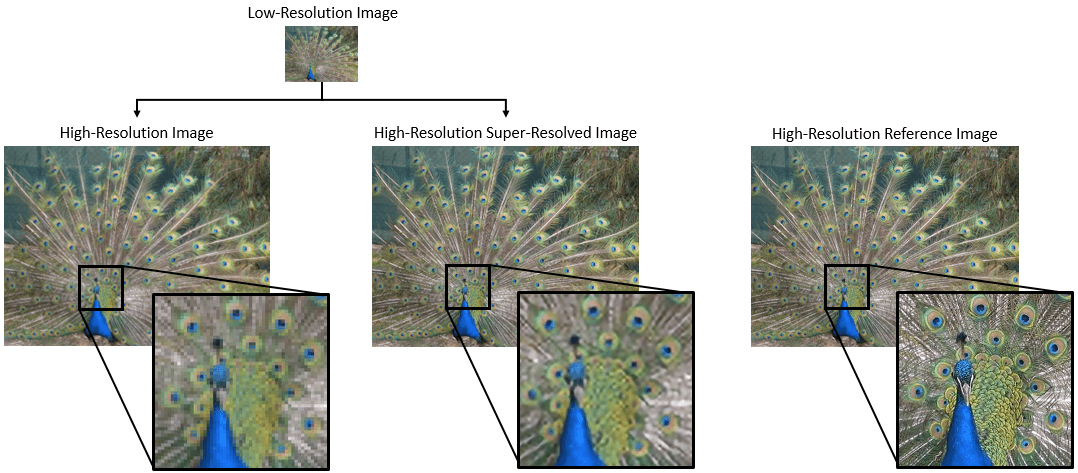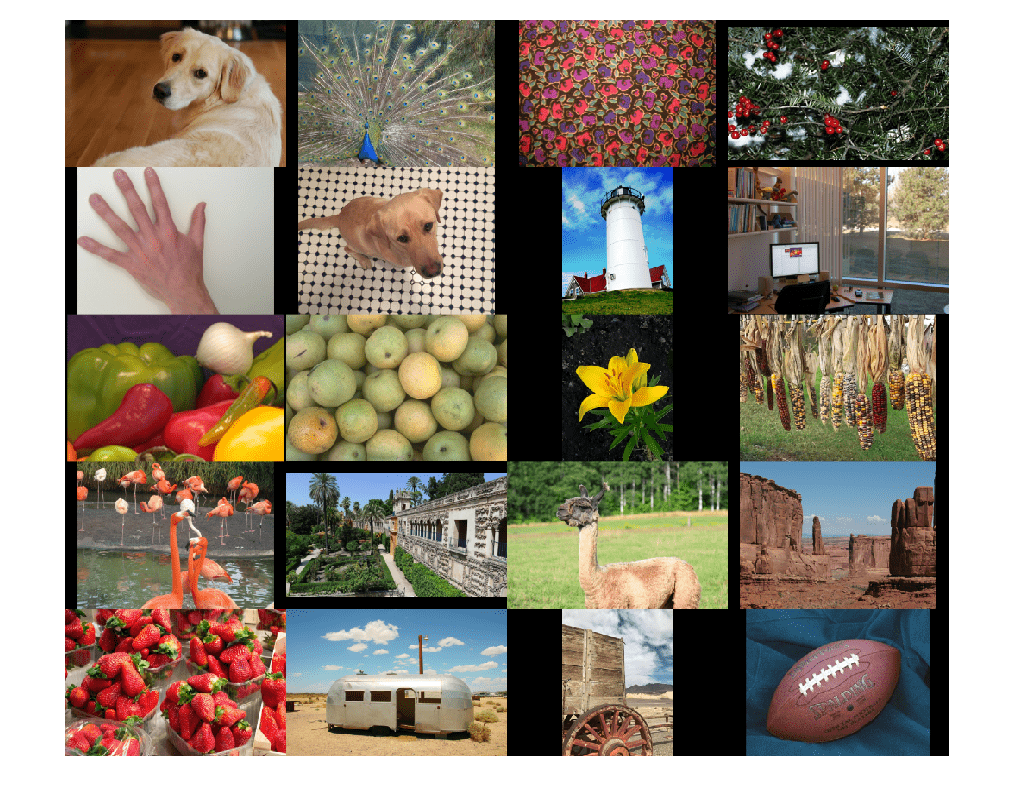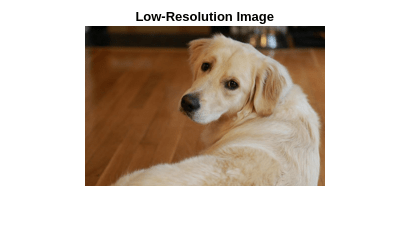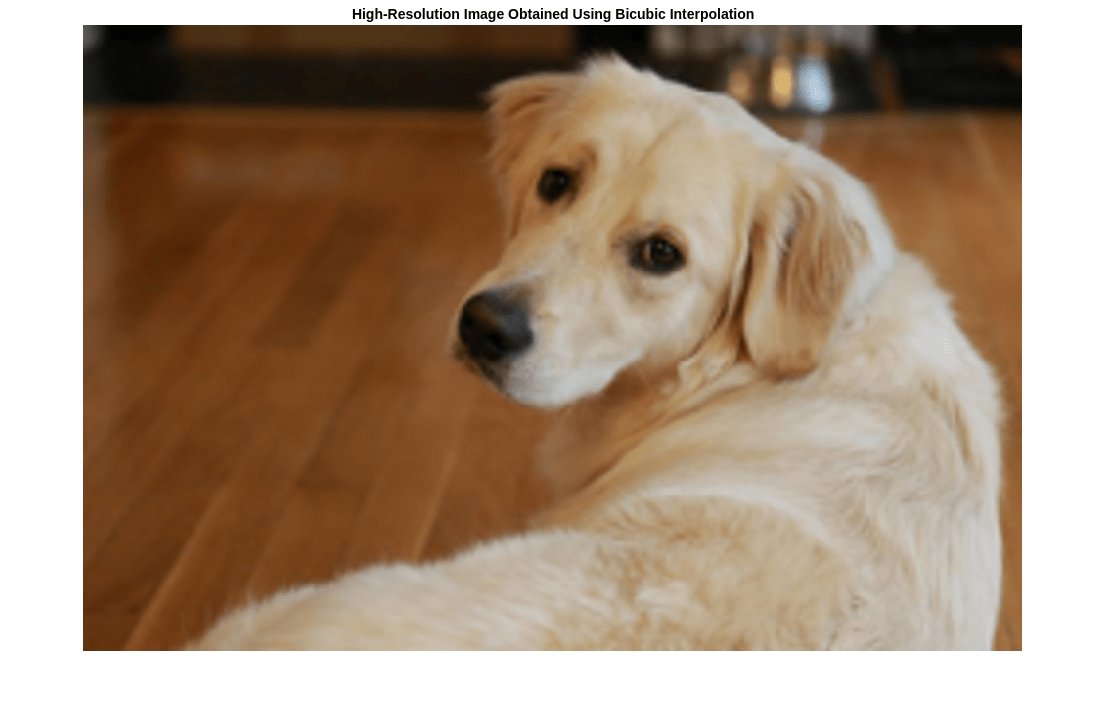# Increase Image Resolution Using Deep Learning

This example shows how to create a high-resolution image from a low-resolution image using a very-deep super-resolution (VDSR) neural network.

Super-resolution is the process of creating high-resolution images from low-resolution images. This example considers single image super-resolution (SISR), where the goal is to recover one high-resolution image from one low-resolution image. SISR is challenging because high-frequency image content typically cannot be recovered from the low-resolution image. Without high-frequency information, the quality of the high-resolution image is limited. Further, SISR is an ill-posed problem because one low-resolution image can yield several possible high-resolution images.Several techniques, including deep learning algorithms, have been proposed to perform SISR. This example explores one deep learning algorithm for SISR, called very-deep super-resolution (VDSR) .

### The VDSR Network

VDSR is a convolutional neural network architecture designed to perform single image super-resolution . The VDSR network learns the mapping between low- and high-resolution images. This mapping is possible because low-resolution and high-resolution images have similar image content and differ primarily in high-frequency details.

VDSR employs a residual learning strategy, meaning that the network learns to estimate a residual image. In the context of super-resolution, a residual image is the difference between a high-resolution reference image and a low-resolution image that has been upscaled using bicubic interpolation to match the size of the reference image. A residual image contains information about the high-frequency details of an image.

The VDSR network detects the residual image from the luminance of a color image. The luminance channel of an image, `Y`, represents the brightness of each pixel through a linear combination of the red, green, and blue pixel values. In contrast, the two chrominance channels of an image, Cb and Cr, are different linear combinations of the red, green, and blue pixel values that represent color-difference information. VDSR is trained using only the luminance channel because human perception is more sensitive to changes in brightness than to changes in color.If ${\mathit{Y}}_{\mathrm{high}\mathrm{res}}$ is the luminance of the high-resolution image and ${\mathit{Y}}_{\mathrm{lowres}}$ is the luminance a low-resolution image that has been upscaled using bicubic interpolation, then the input to the VDSR network is ${\mathit{Y}}_{\mathrm{lowres}}$ and the network learns to predict ${\mathit{Y}}_{\mathrm{residual}}\text{\hspace{0.17em}}=\text{\hspace{0.17em}}{\mathit{Y}}_{\mathrm{highres}}-{\mathit{Y}}_{\mathrm{lowres}}$ from the training data.

After the VDSR network learns to estimate the residual image, you can reconstruct high-resolution images by adding the estimated residual image to the upsampled low-resolution image, then converting the image back to the RGB color space.

A scale factor relates the size of the reference image to the size of the low-resolution image. As the scale factor increases, SISR becomes more ill-posed because the low-resolution image loses more information about the high-frequency image content. VDSR solves this problem by using a large receptive field. This example trains a VDSR network with multiple scale factors using scale augmentation. Scale augmentation improves the results at larger scale factors because the network can take advantage of the image context from smaller scale factors. Additionally, the VDSR network can generalize to accept images with noninteger scale factors.

Download the IAPR TC-12 Benchmark, which consists of 20,000 still natural images . The data set includes photos of people, animals, cities, and more. The size of the data file is ~1.8 GB. If you do not want to download the training data set, then you can load the pretrained VDSR network by typing `load("trainedVDSRNet.mat");` at the command line. Then, go directly to the Perform Single Image Super-Resolution Using VDSR Network section in this example.

Use the helper function, `downloadIAPRTC12Data`, to download the data. This function is attached to the example as a supporting file. Specify `dataDir` as the desired location of the data.

```dataDir =tempdir; downloadIAPRTC12Data(dataDir);```

This example will train the network with a small subset of the IAPR TC-12 Benchmark data. Load the imageCLEF training data. All images are 32-bit JPEG color images.

```trainImagesDir = fullfile(dataDir,"iaprtc12","images","02"); exts = [".jpg",".bmp",".png"]; pristineImages = imageDatastore(trainImagesDir,FileExtensions=exts);```

List the number of training images.

`numel(pristineImages.Files)`
```ans = 616 ```

### Prepare Training Data

To create a training data set, generate pairs of images consisting of upsampled images and the corresponding residual images.

The upsampled images are stored on disk as MAT files in the directory `upsampledDirName`. The computed residual images representing the network responses are stored on disk as MAT files in the directory `residualDirName`. The MAT files are stored as data type `double` for greater precision when training the network.

```upsampledDirName = trainImagesDir+filesep+"upsampledImages"; residualDirName = trainImagesDir+filesep+"residualImages";```

Use the helper function `createVDSRTrainingSet` to preprocess the training data. This function is attached to the example as a supporting file.

The helper function performs these operations for each pristine image in `trainImages`:

• Convert the image to the YCbCr color space

• Downsize the luminance (Y) channel by different scale factors to create sample low-resolution images, then resize the images to the original size using bicubic interpolation

• Calculate the difference between the pristine and resized images.

• Save the resized and residual images to disk.

```scaleFactors = [2 3 4]; createVDSRTrainingSet(pristineImages,scaleFactors,upsampledDirName,residualDirName);```

### Define Preprocessing Pipeline for Training Set

In this example, the network inputs are low-resolution images that have been upsampled using bicubic interpolation. The desired network responses are the residual images. Create an image datastore called `upsampledImages` from the collection of input image files. Create an image datastore called `residualImages` from the collection of computed residual image files. Both datastores require a helper function, `matRead`, to read the image data from the image files. This function is attached to the example as a supporting file.

```upsampledImages = imageDatastore(upsampledDirName,FileExtensions=".mat",ReadFcn=@matRead); residualImages = imageDatastore(residualDirName,FileExtensions=".mat",ReadFcn=@matRead);```

Create an `imageDataAugmenter` that specifies the parameters of data augmentation. Use data augmentation during training to vary the training data, which effectively increases the amount of available training data. Here, the augmenter specifies random rotation by 90 degrees and random reflections in the x-direction.

```augmenter = imageDataAugmenter( ... RandRotatio=@()randi([0,1],1)*90, ... RandXReflection=true);```

Create a `randomPatchExtractionDatastore` (Image Processing Toolbox) that performs randomized patch extraction from the upsampled and residual image datastores. Patch extraction is the process of extracting a large set of small image patches, or tiles, from a single larger image. This type of data augmentation is frequently used in image-to-image regression problems, where many network architectures can be trained on very small input image sizes. This means that a large number of patches can be extracted from each full-sized image in the original training set, which greatly increases the size of the training set.

```patchSize = [41 41]; patchesPerImage = 64; dsTrain = randomPatchExtractionDatastore(upsampledImages,residualImages,patchSize, ... DataAugmentation=augmenter,PatchesPerImage=patchesPerImage);```

The resulting datastore, `dsTrain`, provides mini-batches of data to the network at each iteration of the epoch. Preview the result of reading from the datastore.

```inputBatch = preview(dsTrain); disp(inputBatch)```
``` InputImage ResponseImage ______________ ______________ {41×41 double} {41×41 double} {41×41 double} {41×41 double} {41×41 double} {41×41 double} {41×41 double} {41×41 double} {41×41 double} {41×41 double} {41×41 double} {41×41 double} {41×41 double} {41×41 double} {41×41 double} {41×41 double} ```

### Set Up VDSR Layers

This example defines the VDSR network using 41 individual layers from Deep Learning Toolbox™, including:

The first layer, `imageInputLayer`, operates on image patches. The patch size is based on the network receptive field, which is the spatial image region that affects the response of the top-most layer in the network. Ideally, the network receptive field is the same as the image size so that the field can see all the high-level features in the image. In this case, for a network with D convolutional layers, the receptive field is (2D+1)-by-(2D+1).

VDSR has 20 convolutional layers so the receptive field and the image patch size are 41-by-41. The image input layer accepts images with one channel because VDSR is trained using only the luminance channel.

```networkDepth = 20; firstLayer = imageInputLayer([41 41 1],Name="InputLayer",Normalization="none");```

The image input layer is followed by a 2-D convolutional layer that contains 64 filters of size 3-by-3. The mini-batch size determines the number of filters. Zero-pad the inputs to each convolutional layer so that the feature maps remain the same size as the input after each convolution. He's method  initializes the weights to random values so that there is asymmetry in neuron learning. Each convolutional layer is followed by a ReLU layer, which introduces nonlinearity in the network.

```convLayer = convolution2dLayer(3,64,Padding=1, ... WeightsInitializer="he",BiasInitializer="zeros",Name="Conv1");```

Specify a ReLU layer.

`relLayer = reluLayer(Name="ReLU1");`

The middle layers contain 18 alternating convolutional and rectified linear unit layers. Every convolutional layer contains 64 filters of size 3-by-3-by-64, where a filter operates on a 3-by-3 spatial region across 64 channels. As before, a ReLU layer follows every convolutional layer.

```middleLayers = [convLayer relLayer]; for layerNumber = 2:networkDepth-1 convLayer = convolution2dLayer(3,64,Padding=[1 1], ... WeightsInitializer="he",BiasInitializer="zeros", ... Name="Conv"+num2str(layerNumber)); relLayer = reluLayer(Name="ReLU"+num2str(layerNumber)); middleLayers = [middleLayers convLayer relLayer]; end```

The penultimate layer is a convolutional layer with a single filter of size 3-by-3-by-64 that reconstructs the image.

```convLayer = convolution2dLayer(3,1,Padding=[1 1], ... WeightsInitializer="he",BiasInitializer="zeros", ... NumChannels=64,Name="Conv"+num2str(networkDepth));```

The last layer is a regression layer instead of a ReLU layer. The regression layer computes the mean-squared error between the residual image and network prediction.

`finalLayers = [convLayer regressionLayer(Name="FinalRegressionLayer")];`

Concatenate all the layers to form the VDSR network.

`layers = [firstLayer middleLayers finalLayers];`

### Specify Training Options

Train the network using stochastic gradient descent with momentum (SGDM) optimization. Specify the hyperparameter settings for SGDM by using the `trainingOptions` function. The learning rate is initially `0.1` and decreased by a factor of 10 every 10 epochs. Train for 100 epochs.

Training a deep network is time-consuming. Accelerate the training by specifying a high learning rate. However, this can cause the gradients of the network to explode or grow uncontrollably, preventing the network from training successfully. To keep the gradients in a meaningful range, enable gradient clipping by specifying "`GradientThreshold"` as `0.01`, and specify "`GradientThresholdMethod"` to use the L2-norm of the gradients.

```maxEpochs = 100; epochIntervals = 1; initLearningRate = 0.1; learningRateFactor = 0.1; l2reg = 0.0001; miniBatchSize = 64; options = trainingOptions("sgdm", ... Momentum=0.9, ... InitialLearnRate=initLearningRate, ... LearnRateSchedule="piecewise", ... LearnRateDropPeriod=10, ... LearnRateDropFactor=learningRateFactor, ... L2Regularization=l2reg, ... MaxEpochs=maxEpochs, ... MiniBatchSize=miniBatchSize, ... GradientThresholdMethod="l2norm", ... GradientThreshold=0.01, ... Plots="training-progress", ... Verbose=false);```

### Train the Network

By default, the example loads a pretrained version of the VDSR network that has been trained to super-resolve images for scale factors 2, 3 and 4. The pretrained network enables you to perform super-resolution of test images without waiting for training to complete.

To train the VDSR network, set the `doTraining` variable in the following code to `true`. Train the network using the `trainNetwork` function.

Train on a GPU if one is available. Using a GPU requires Parallel Computing Toolbox™ and a CUDA® enabled NVIDIA® GPU. For more information, see GPU Computing Requirements (Parallel Computing Toolbox). Training takes about 6 hours on an NVIDIA Titan X.

```doTraining =false; if doTraining net = trainNetwork(dsTrain,layers,options); modelDateTime = string(datetime("now",Format="yyyy-MM-dd-HH-mm-ss")); save("trainedVDSR-"+modelDateTime+".mat","net"); else load("trainedVDSRNet.mat"); end```

### Perform Single Image Super-Resolution Using VDSR Network

To perform single image super-resolution (SISR) using the VDSR network, follow the remaining steps of this example:

• Create a sample low-resolution image from a high-resolution reference image.

• Perform SISR on the low-resolution image using bicubic interpolation, a traditional image processing solution that does not rely on deep learning.

• Perform SISR on the low-resolution image using the VDSR neural network.

• Visually compare the reconstructed high-resolution images using bicubic interpolation and VDSR.

• Evaluate the quality of the super-resolved images by quantifying the similarity of the images to the high-resolution reference image.

### Create Sample Low-Resolution Image

The test data set, `testImages`, contains 20 undistorted images shipped in Image Processing Toolbox™. Load the images into an `imageDatastore` and display the images in a montage.

```fileNames = ["sherlock.jpg","peacock.jpg","fabric.png","greens.jpg", ... "hands1.jpg","kobi.png","lighthouse.png","office_4.jpg", ... "onion.png","pears.png","yellowlily.jpg","indiancorn.jpg", ... "flamingos.jpg","sevilla.jpg","llama.jpg","parkavenue.jpg", ... "strawberries.jpg","trailer.jpg","wagon.jpg","football.jpg"]; filePath = fullfile(matlabroot,"toolbox","images","imdata")+filesep; filePathNames = strcat(filePath,fileNames); testImages = imageDatastore(filePathNames);```

Display the test images as a montage.

`montage(testImages)`Select one of the test images to use for testing the super-resolution network.

```testImage ="sherlock.jpg"; Ireference = imread(testImage); Ireference = im2double(Ireference); imshow(Ireference) title("High-Resolution Reference Image")```Create a low-resolution version of the high-resolution reference image by using `imresize` with a scaling factor of 0.25. The high-frequency components of the image are lost during the downscaling.

```scaleFactor = 0.25; Ilowres = imresize(Ireference,scaleFactor,"bicubic"); imshow(Ilowres) title("Low-Resolution Image")```### Improve Image Resolution Using Bicubic Interpolation

A standard way to increase image resolution without deep learning is to use bicubic interpolation. Upscale the low-resolution image using bicubic interpolation so that the resulting high-resolution image is the same size as the reference image.

```[nrows,ncols,np] = size(Ireference); Ibicubic = imresize(Ilowres,[nrows ncols],"bicubic"); imshow(Ibicubic) title("High-Resolution Image Obtained Using Bicubic Interpolation")```### Improve Image Resolution Using Pretrained VDSR Network

Recall that VDSR is trained using only the luminance channel of an image because human perception is more sensitive to changes in brightness than to changes in color.

Convert the low-resolution image from the RGB color space to luminance (`Iy`) and chrominance (`Icb` and `Icr`) channels by using the `rgb2ycbcr` (Image Processing Toolbox) function.

```Iycbcr = rgb2ycbcr(Ilowres); Iy = Iycbcr(:,:,1); Icb = Iycbcr(:,:,2); Icr = Iycbcr(:,:,3);```

Upscale the luminance and two chrominance channels using bicubic interpolation. The upsampled chrominance channels, `Icb_bicubic` and `Icr_bicubic`, require no further processing.

```Iy_bicubic = imresize(Iy,[nrows ncols],"bicubic"); Icb_bicubic = imresize(Icb,[nrows ncols],"bicubic"); Icr_bicubic = imresize(Icr,[nrows ncols],"bicubic");```

Pass the upscaled luminance component, `Iy_bicubic`, through the trained VDSR network. Observe the `activations` from the final layer (a regression layer). The output of the network is the desired residual image.

```Iresidual = activations(net,Iy_bicubic,41); Iresidual = double(Iresidual); imshow(Iresidual,[]) title("Residual Image from VDSR")```Add the residual image to the upscaled luminance component to get the high-resolution VDSR luminance component.

`Isr = Iy_bicubic + Iresidual;`

Concatenate the high-resolution VDSR luminance component with the upscaled color components. Convert the image to the RGB color space by using the `ycbcr2rgb` (Image Processing Toolbox) function. The result is the final high-resolution color image using VDSR.

```Ivdsr = ycbcr2rgb(cat(3,Isr,Icb_bicubic,Icr_bicubic)); imshow(Ivdsr) title("High-Resolution Image Obtained Using VDSR")```### Visual and Quantitative Comparison

To get a better visual understanding of the high-resolution images, examine a small region inside each image. Specify a region of interest (ROI) using vector `roi` in the format [x y width height]. The elements define the x- and y-coordinate of the top left corner, and the width and height of the ROI.

`roi = [360 50 400 350];`

Crop the high-resolution images to this ROI, and display the result as a montage. The VDSR image has clearer details and sharper edges than the high-resolution image created using bicubic interpolation.

```montage({imcrop(Ibicubic,roi),imcrop(Ivdsr,roi)}) title("High-Resolution Results Using Bicubic Interpolation (Left) vs. VDSR (Right)");```Use image quality metrics to quantitatively compare the high-resolution image using bicubic interpolation to the VDSR image. The reference image is the original high-resolution image, `Ireference`, before preparing the sample low-resolution image.

Measure the peak signal-to-noise ratio (PSNR) of each image against the reference image. Larger PSNR values generally indicate better image quality. See `psnr` (Image Processing Toolbox) for more information about this metric.

`bicubicPSNR = psnr(Ibicubic,Ireference)`
```bicubicPSNR = 38.4747 ```
`vdsrPSNR = psnr(Ivdsr,Ireference)`
```vdsrPSNR = 39.2346 ```

Measure the structural similarity index (SSIM) of each image. SSIM assesses the visual impact of three characteristics of an image: luminance, contrast and structure, against a reference image. The closer the SSIM value is to 1, the better the test image agrees with the reference image. See `ssim` (Image Processing Toolbox) for more information about this metric.

`bicubicSSIM = ssim(Ibicubic,Ireference)`
```bicubicSSIM = 0.9861 ```
`vdsrSSIM = ssim(Ivdsr,Ireference)`
```vdsrSSIM = 0.9874 ```

Measure perceptual image quality using the Naturalness Image Quality Evaluator (NIQE). Smaller NIQE scores indicate better perceptual quality. See `niqe` (Image Processing Toolbox) for more information about this metric.

`bicubicNIQE = niqe(Ibicubic)`
```bicubicNIQE = 5.1721 ```
`vdsrNIQE = niqe(Ivdsr)`
```vdsrNIQE = 4.7612 ```

Calculate the average PSNR and SSIM of the entire set of test images for the scale factors 2, 3, and 4. For simplicity, you can use the helper function, `vdsrMetrics`, to compute the average metrics. This function is attached to the example as a supporting file.

```scaleFactors = [2 3 4]; vdsrMetrics(net,testImages,scaleFactors);```
```Results for Scale factor 2 Average PSNR for Bicubic = 31.467070 Average PSNR for VDSR = 31.481973 Average SSIM for Bicubic = 0.935820 Average SSIM for VDSR = 0.947057 Results for Scale factor 3 Average PSNR for Bicubic = 28.107057 Average PSNR for VDSR = 28.430546 Average SSIM for Bicubic = 0.883927 Average SSIM for VDSR = 0.894634 Results for Scale factor 4 Average PSNR for Bicubic = 27.066129 Average PSNR for VDSR = 27.846590 Average SSIM for Bicubic = 0.863270 Average SSIM for VDSR = 0.878101 ```

VDSR has better metric scores than bicubic interpolation for each scale factor.

### References

 Kim, J., J. K. Lee, and K. M. Lee. "Accurate Image Super-Resolution Using Very Deep Convolutional Networks." Proceedings of the IEEE® Conference on Computer Vision and Pattern Recognition. 2016, pp. 1646-1654.

 Grubinger, M., P. Clough, H. Müller, and T. Deselaers. "The IAPR TC-12 Benchmark: A New Evaluation Resource for Visual Information Systems." Proceedings of the OntoImage 2006 Language Resources For Content-Based Image Retrieval. Genoa, Italy. Vol. 5, May 2006, p. 10.

 He, K., X. Zhang, S. Ren, and J. Sun. "Delving Deep into Rectifiers: Surpassing Human-Level Performance on ImageNet Classification." Proceedings of the IEEE International Conference on Computer Vision, 2015, pp. 1026-1034.